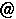### Solutions to problems of the problem list " Problems on Invariants of Knots and 3-Manifolds"

Problem 1.15:
H. Gruber showed the following results toward a solution of this problem.
- If the statement of this problem is true for all prime knots, then it is true for all knots.
- The statement is true for all alternating knots whose Conway basic polyhedron has odd chromatic invariant. In particular, the statement is true for all algebraic alternating knots.
See [Gruber, Math. Proc. Camb. Phil. Soc. 147 (2009)].

Conjecture 5.3:
M. Eisermann showed that this conjecture is true. Since h_X(K) is bounded by |X|^(braid index of K), the conjecture is obtained from Theorem 3 of [ Eisermann, Proc. Amer. Math. Soc. 128 (2000)].

Problem 12.17:
This problem has been solved by Budney. The homotopy type of the space of long knots with a fixed knot type can be calculated by reducing it to the homotopy types of the spaces of long knots with shorter companionship trees; see [Budney, math.GT/0506524].

(Partial) solutions, comments or suggestions to problems of the problem list would be welcome.

tomotadakurims.kyoto-u.ac.jp